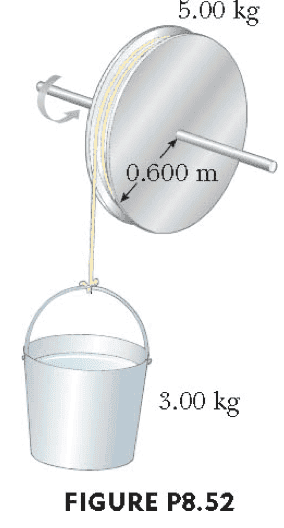# Final angular velocity of a mass on a pulley

austindubose

## Homework Statement

Use Newton's 2nd Law, sum of torques, and kinematic equations to determine the angular speed of the spool shown in the figure below. Assume the string has a negligible mass, and it turns without slipping. Use g=10 m/s2 for acceleration due to gravity.Ʃτ=rxF=Iα
F=mA
ωf2i2+2αΔθ

## The Attempt at a Solution

Sum of torques:
Ʃτ=rxf
=(.6m)(3kg*10m/s2)(sin 90°)=Iα
--> 18 kgm2/s2=Iα
α=18/[.5(5)(.62)]